Hi Mapleprimes,

bye

## Feynman Integrals

by: MapleEdgardo S. Cheb-Terrab
Physics, Differential Equations and Mathematical Functions, Maplesoft

## Old bug in `diff/abs`

by: Maple

diff(abs(z), z)  returns abs(1, z)  and the latter, for a numeric z, is defined only for a nonzero real z.
However,  functions such as abs(I+sin(t)) are (real) differentiable for a real t and diff should work. It usually does, but not always.

```restart
f:= t -> abs(GAMMA(2*t+I)):  # We want D(f)(1)
D(f)(1):
evalf(%);  # Error, (in simpl/abs) abs is not differentiable at non-real arguments
D(f)(1); simplify(%);
evalf(%);   # 0.3808979508 + 1.161104935*I,  wrong
```

The same wrong results are obtained with diff instead of D

```diff(f(t),t):   # or  diff(f(t),t) assuming t::real;
eval(%,t=1);
simplify(%); evalf(%);   # wrong, should be real
```

To obtain the correct result, we could use the definition of the derivative:

```limit((f(t)-f(1))/(t-1), t=1); evalf(%); # OK
fdiff(f(t), t=1);    # numeric, OK
```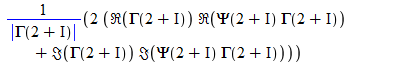0.8772316598
0.8772316599

Note that abs(1, GAMMA(2 + I)); returns 1; this is also wrong, it should produce an error because  GAMMA(2+I) is not real;

Let's redefine now `diff/abs`  and redo the computations.

```restart
`diff/abs` := proc(u, z)   # implements d/dx |f(x+i*y|) when f is analytic and f(...)<>0
local u1:=diff(u,z);
1/abs(u)*( Re(u)*Re(u1) + Im(u)*Im(u1) )
end:
f:= t -> abs(GAMMA(2*t+I));
D(f)(1); evalf(%);   # OK now
```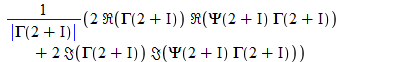0.8772316597

Now diff works too.

```diff(f(t),t);
eval(%,t=1);
simplify(%); evalf(%);   # it works
```

This is a probably a very old bug which may make diff(u,x)  fail for expressions having subespressions abs(...) depending on x.

However it works  using assuming x::real, but only if evalc simplifies u.
The problem is actually more serious because diff/ for Re, Im, conjugate should be revized too. Plus some other related things.

## Putnam Mathematical Competition 2020

by: Maple

The Putnam 2020 Competition (the 81st) was postponed to February 20, 2021 due to the COVID-19 pandemic, and held in an unofficial mode with no prizes or official results.

Four of the problems have surprisingly short Maple solutions.
Here they are.

A1.  How many positive integers N satisfy all of the following three conditions?
(i) N is divisible by 2020.
(ii) N has at most 2020 decimal digits.
(iii) The decimal digits of N are a string of consecutive ones followed by a string of consecutive zeros.

```add(add(`if`( (10&^m-1)*10&^(n-m) mod 2020 = 0, 1, 0),
n=m+1..2020), m=1..2020);
```

508536

A2.  Let k be a nonnegative integer.  Evaluate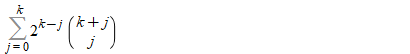```sum(2^(k-j)*binomial(k+j,j), j=0..k);
```

4^k

A3.  Let a(0) = π/2, and let a(n) = sin(a(n-1)) for n ≥ 1.
Determine whether the series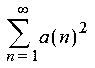converges.

```asympt('rsolve'({a(n) = sin(a(n-1)),a(0)=Pi/2}, a(n)), n, 4);
```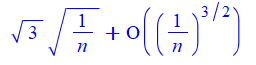a(n) ^2 being equivalent to 3/n,  the series diverges.

B1.  For a positive integer n, define d(n) to be the sum of the digits of n when written in binary
(for example, d(13) = 1+1+0+1 = 3).

Let   S =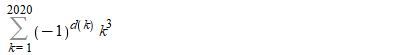Determine S modulo 2020.

```d := n -> add(convert(n, base,2)):
add( (-1)^d(k) * k^3, k=1..2020 ) mod 2020;
```

1990

## Vibrational Mechanics - Practical Applications

by: MaplePresenters:

Physics Department, Northeastern University

United States of America

&

School of Advanced Manufacturing & Mechanical Engineering

University of South Australia

Australia

Note: This webinar is free. For registration, please send an e-mail to m.khoshnevisan@ieee.org. The registration link will be sent to all the participants on April 26, 2021. Maximum number of participants =60

Vibrational Mechanics - Practical Applications- Animation 1

Vibrational Mechanics - Practical Applications- Animation 2

Vibrational Mechanics - Practical Applications- Animation 3

by: Maple

## Maple Calculator – now with steps!

by: Maple Calculator

I’m very pleased to announce that the Maple Calculator app now offers step-by-step solutions. Maple Calculator is a free mobile app that makes it easy to enter, solve, and visualize mathematical problems from algebra, precalculus, calculus, linear algebra, and differential equations, right on your phone.  Solution steps have been, by far, the most requested feature from Maple Calculator users, so we are pretty excited about being able to offer this functionality to our customers. With steps, students can use the app not just to check if their own work is correct, but to find the source of the problem if they made a mistake.  They can also use the steps to learn how to approach problems they are unfamiliar with.

Steps are available in Maple Calculator for a wide variety of problems, including solving equations and systems of equations, finding limits, derivatives, and integrals, and performing matrix operations such as finding inverses and eigenvalues.

(*Spoiler alert* You may also want to keep a look-out for more step-by-step solution abilities in the next Maple release.)

If you are unfamiliar with the Maple Calculator app, you can find more information and app store links on the Maple Calculator product page.  One feature in particular to note for Maple and Maple Learn users is that you can use the app to take a picture of your math and load those math expressions into Maple or Maple Learn.  It makes for a fast, accurate method for entering large expressions, so even if you aren’t interested in doing math on your phone, you still might find the app useful.

## Pythagorean Triples Ternary Tree

Maple 18

I make a maple worksheet for generating Pythagorean Triples Ternary Tree :

Around 10,000 records in the matrix currently !

You can set your desire size or export the Matrix as text ...

But yet ! I wish to understand from you better techniques If you have some suggestion ?

the mapleprimes Don't load my worksheet for preview so i put a screenshot !Pythagoras_ternary.mw

Pythagoras_ternary_data.mw

Pythagoras_ternary_maple.mw

## New book Fourier Transforms for Chemistry

by: Maple 2020

We announce the release of a new book, of title Fourier Transforms for Chemistry, which is in the form of a Maple worksheet.  This book is freely available through Maple Application Centre, either as a Maple worksheet with no output from commands or as a .pdf file with all output and plots.

This interactive electronic book in the form of a Maple worksheet comprises six chapters containing Maple commands, plus an overview 0 as an introduction.  The chapters have content as follows.

-   1    continuous Fourier transformation

-   2    electron diffraction of a gaseous sample

-   3    xray diffraction of a crystal and a powder

-   4    microwave spectrum of a gaseous sample

-   5    infrared and Raman spectra of a liquid sample

-   6    nuclear magnetic resonance of various samples

This book will be useful in courses of physical chemistry or devoted to the determination of molecular structure by physical methods.  Some content, duly acknowledged, has been derived and adapted from other authors, with permission.

## pillow curve

Maple 13

Hi all,

Look at my pretty plot.  It is defined by

x=sin(m*t);
y=sin(n*t);

where n and m are one digit positive integers.

You can modify my worksheet with different values of n and m.

pillow_curve.mw

pillow_curve.pdf

The name of the curve may be something like Curve of Lesotho.  I saw this first in one of my father's books.

Regards,
Matt

## Document attachments using links in MaplePrimes...

by: MaplePrimes

The above doc/worksheet can be clicked and viewed.

Youtube URL is given below. This also can be clicked and viewed. Thanks to Carl Love's suggestion, I modified using the chain link in the editor.

https://youtu.be/0pDa4FWMSQo

## Dark Theme For Maple

by:

Hello Congratulations on the great product! Isn't time time Maple got a dark theme for us night owls ! Thanx

## Maple Evaluation Order Example

by: Maple 2020

The order in which expressions evaluate in Maple is something that occasionally causes even advanced users to make syntax errors.

I recently saw a single line of Maple code that provided a good example of a command not evaluating in the order the user desired.

The command in question (after calling with(plots):) was

`animate(display, [arrow(<cos(t), sin(t)>)], t = 0 .. 2*Pi)`

This resulted in the error:

Error, (in plots/arrow) invalid input: plottools:-arrow expects its 3rd argument, pv, to be of type {Vector, list, vector, complexcons, realcons}, but received 0.5000000000e-1*(cos(t)^2+sin(t)^2)^(1/2)

This error indicates that the issue in the animation is the arrow command

`arrow(<cos(t), sin(t)>)`

on its own, the above command would give the same error. However, the animate command takes values of t from 0 to 2*Pi and substitutes them in, so at first glance, you wouldn't expect the same error to occur.

What is happening is that the command

`arrow(<cos(t), sin(t)>)`

in the animate expression is evaluating fully, BEFORE the call to animate is happening. This is due to Maple's automatic simplification (since if this expression contained large expressions, or the values were calculated from other function calls, that could be simplified down, you'd want that to happen first to prevent unneeded calculation time at each step).

So the question is how do we stop it evaluating ahead of time since that isn't what we want to happen in this case?

In order to do this we can use uneval quotes (the single quotes on the keyboard - not to be confused with the backticks).

`animate(display, ['arrow'(<cos(t), sin(t)>)], t = 0 .. 2*Pi)`

By surrounding the arrow function call in the uneval quotes, the evaluation is delayed by a level, allowing the animate call to happen first so that each value of t is then substituted before the arrow command is called.

Maple_Evaluation_Order_Example.mw

## pendulum animation: decomposing a vector into...

by: Maple

Most of the time in multivariable calculus the vectors we are decomposing with respect to a given direction are attached to a specific point and this "orthogonal decomposition" should be visualized as a triplet of vectors with initial point at that given point such that the two projections sum to the original vector. Keep in mind our high school physics pendulum example where we need to evaluate both the component of the downward gravitational force along the tangential direction of motion of the pendulum for the equations of motion as well as along the perpendicular direction to the motion to evaluate the tension in the string or wire holding the pendulum mass.

We create an Explorable graphic to visualize this idea and then create an animation which requires a bit of Maple expert user knowledge to accomplish (thanks Maplesoft!). A delayed evaluation of the arrow command from the plot package is required in order to animate that arrow so that it waits for the animation command to feed it actual numerical values for the vector to be plotted.

My worksheet URL:
http://www34.homepage.villanova.edu/robert.jantzen/courses/mat2500/handouts/pendulumprojection.mw

## One more way of inverse kinematics

by:

As a continuation of the posts:
https://www.mapleprimes.com/posts/208958-Determination-Of-The-Angles-Of-The-Manipulator
https://www.mapleprimes.com/posts/209255-The-Use-Of-Manipulators-As-Multiaxis
https://www.mapleprimes.com/posts/210003-Manipulator-With-Variable-Length-Of
But this time without Draghilev's method.
Motion along straight lines can replace motion along any spatial path (with any practical precision), which means that solving the inverse problem of the manipulator's kinematics can be reduced to solving the movement along a sequential set of segments. Thus, another general method for solving the manipulator inverse problem is proposed.
An example of a three-link manipulator with 5 degrees of freedom. Its last link, like the first link, geometrically corresponds to the radius of the sphere. We calculate the coordinates of the ends of its links when passing a straight line segment. We do this in a loop for interior points of the segment using the procedure for finding real roots of polynomial systems of equations RootFinding [Isolate]. First, we “remove” two “extra” degrees of freedom by adding two equations to the system. There can be an infinite set of options for additional equations - if only they correspond to the technical capabilities of the device. In this case, two maximally easy conditions were taken: one equation corresponds to the perpendicularity of the last (third) link directly to the segment of the trajectory itself, and the second equation corresponds to the perpendicularity to the vector with coordinates <1,1,1>. As a result, we got four ways to move the manipulator for the same segment. All of these ways are selected as one of the RootFinding [Isolate] solutions (in text  jj=1,2,3,4).
In this text jj=4
without_Draghilev_method.mw

As you can see, everything is very simple, there is practically no programming and is performed exclusively by Maple procedures.﻿